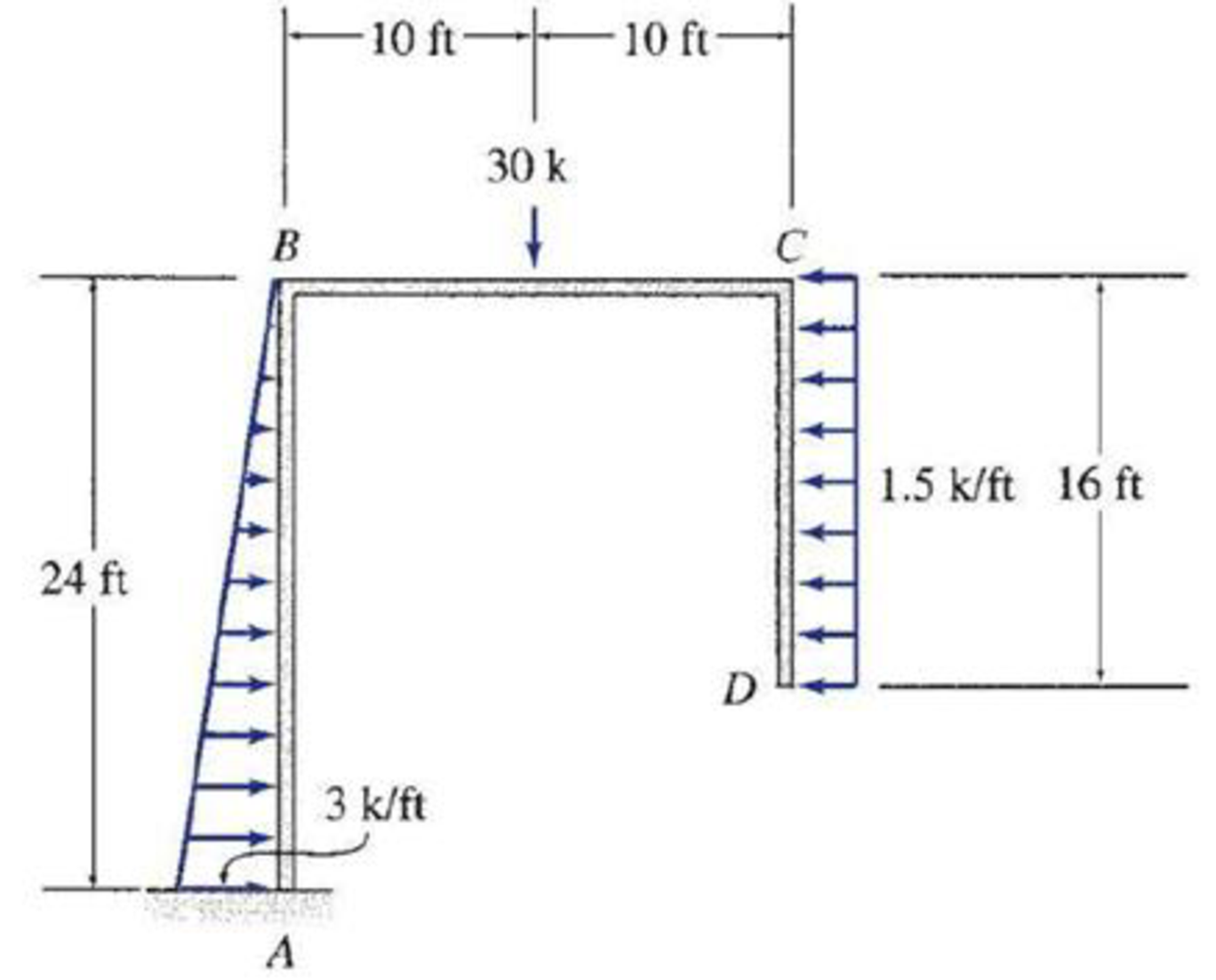# 5.57 through 5.71 Draw the shear, bending moment, and axial force diagrams and the qualitative deflected shape for the frame shown.

#### Solutions

Chapter
Section
Chapter 5, Problem 69P
Textbook Problem
70 views

## 5.57 through 5.71 Draw the shear, bending moment, and axial force diagrams and the qualitative deflected shape for the frame shown.To determine

Plot the shear diagram, bending moment diagram, axial force diagram, and the qualitative deflected shape of the frame.

### Explanation of Solution

Write the condition for static instability, determinacy and indeterminacy of plane frames as follows:

3m+r<3j+ecstaticallyunstableframe        (1)

3m+r=3j+ecstaticallydeterminateframe        (2)

3m+r>3j+ecstaticallyindeterminateframe        (3)

Here, number of members is m, number of external reactions is r, the number of joints is j, and the number of elastic hinges is ec.

Find the degree of static indeterminacy (i) using the equation;

i=(3m+r)(3j+ec)        (4)

Refer to the Figure in the question;

The number of members (m) is 3.

The number of external reactions (r) is 3.

The number of joints (j) is 4.

The number of elastic hinges ec is 0.

Substitute the values in Equation (2);

3(3)+3=3(4)+012=12staticallydeterminateframe

Show the free-body diagram of the entire frame as in Figure 1.

Refer Figure 1,

Find the vertical reaction at point A by resolving the vertical component of forces.

+Fy=0Ay30=0Ay=30k

Find the horizontal reaction at point A by resolving the horizontal component of forces.

+Fx=0Ax+12×3×241.5×16=0Ax+3624=0Ax=12k

Find the moment at point A by taking moment at point A.

+MA=0MA12×3×24×24330(10)+1.5×16×(24162)=0MA288300+384=0MA=204k-ft

Show the free-body diagram of the members and joints of the entire frame as in Figure 2.

Consider point A:

Resolve the vertical component of forces.

+FY=030AYAB=0AYAB=30k

Resolve the horizontal component of forces.

+FX=012+AXAB=0AXAB=12k

Take moment about the point A.

+MAAB=0204MAAB=0MAAB=204k-ft

Consider the member AB:

Resolve the vertical component of forces.

+FY=0AYABBYAB=030BYAB=0BYAB=30k

Resolve the horizontal component of forces.

+FX=0AXAB12×3×24+BXAB=01236+BXAB=0BXAB=24k

Take moment about the point B.

+MBAB=0MBABAXAB(24)+12×3×24×2×243+MAAB=0MBAB12(24)+576+204=0MBAB=492k-ft

Consider the point B:

Resolve the vertical component of forces

### Still sussing out bartleby?

Check out a sample textbook solution.

See a sample solution

#### The Solution to Your Study Problems

Bartleby provides explanations to thousands of textbook problems written by our experts, many with advanced degrees!

Get Started

Find more solutions based on key concepts
For Problem 19.1, calculate the cumulative frequency and plot a cumulative-frequency polygon.

Engineering Fundamentals: An Introduction to Engineering (MindTap Course List)

Explain how enterprise computers use tape for storage.

Enhanced Discovering Computers 2017 (Shelly Cashman Series) (MindTap Course List)

When is a fire watch needed?

Welding: Principles and Applications (MindTap Course List)

If your motherboard supports ECC DDR3 memory, can you substitute non-ECC DDR3 memory?

A+ Guide to Hardware (Standalone Book) (MindTap Course List)